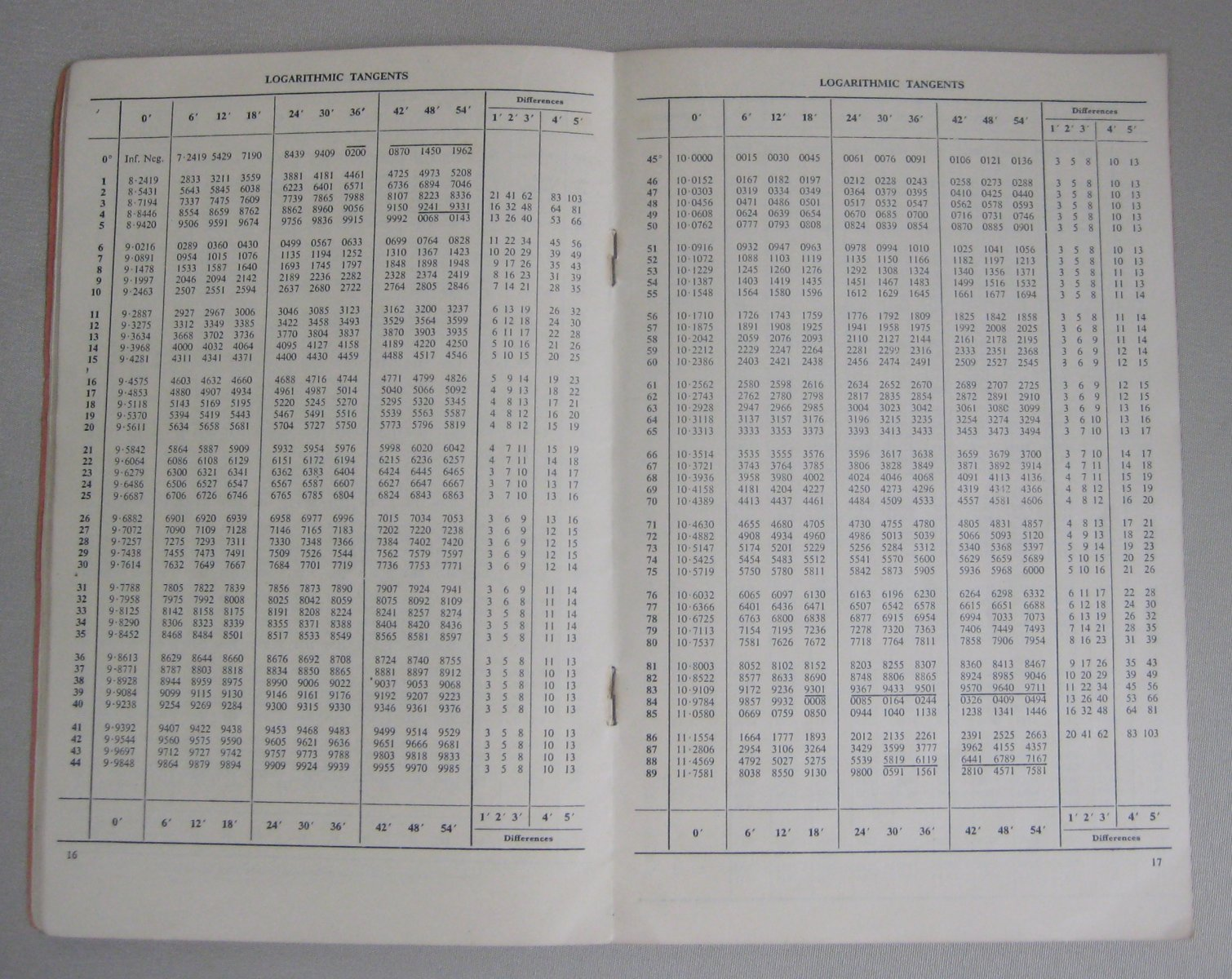ID:
2063
Maker's Name:
various
Tour Audio: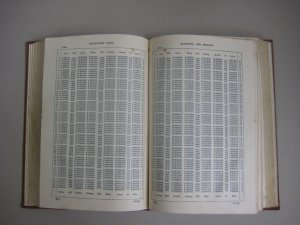Tables of mathematical functions go back to antiquity. The first recorded  mathematical table book was  the  Logarithmorum Chilias Prima published by Henry Briggs and John Napier in 1617. It included the first 1000 logarithms accurate to the 14th decimal place. Since 1617 mathematical tables have been significantly improved and some are still in use today such as normal distribution and cumulative distribution functions.

There are tables for specialised fields such as navigation, astronomy or statistics. More generally however these tables contain lists of values of log⁡(x),x^2,√x,e^x,cosine and other functions. The aim of these tables was to increase the speed of calculations and they were one of the most popular aids before the advent of electronic calculators.

Problems of transcription and translation became evident after ships were reportedly sunk due to navigational errors from tables. Revenue was lost in miscalculations by banks due to mistakes which were not uncommon in the large printed books. In the 1820s this prompted Charles Babbage to design new mechanical methods of calculation to eliminate human errors. While many other mathematical instruments were created, some of which can be viewed in this display, tables remained important until the 1970s.

In the display we have included tables of varying use and length such as a 7 digit book with sine, cosine, natural logarithm and logarithm sections, and a small example sheet explaining how to use the tables. Why don’t you see if you can reach the same answer?

This item is part of the UQ Physics Museum Good Old days of Calculation Tour
•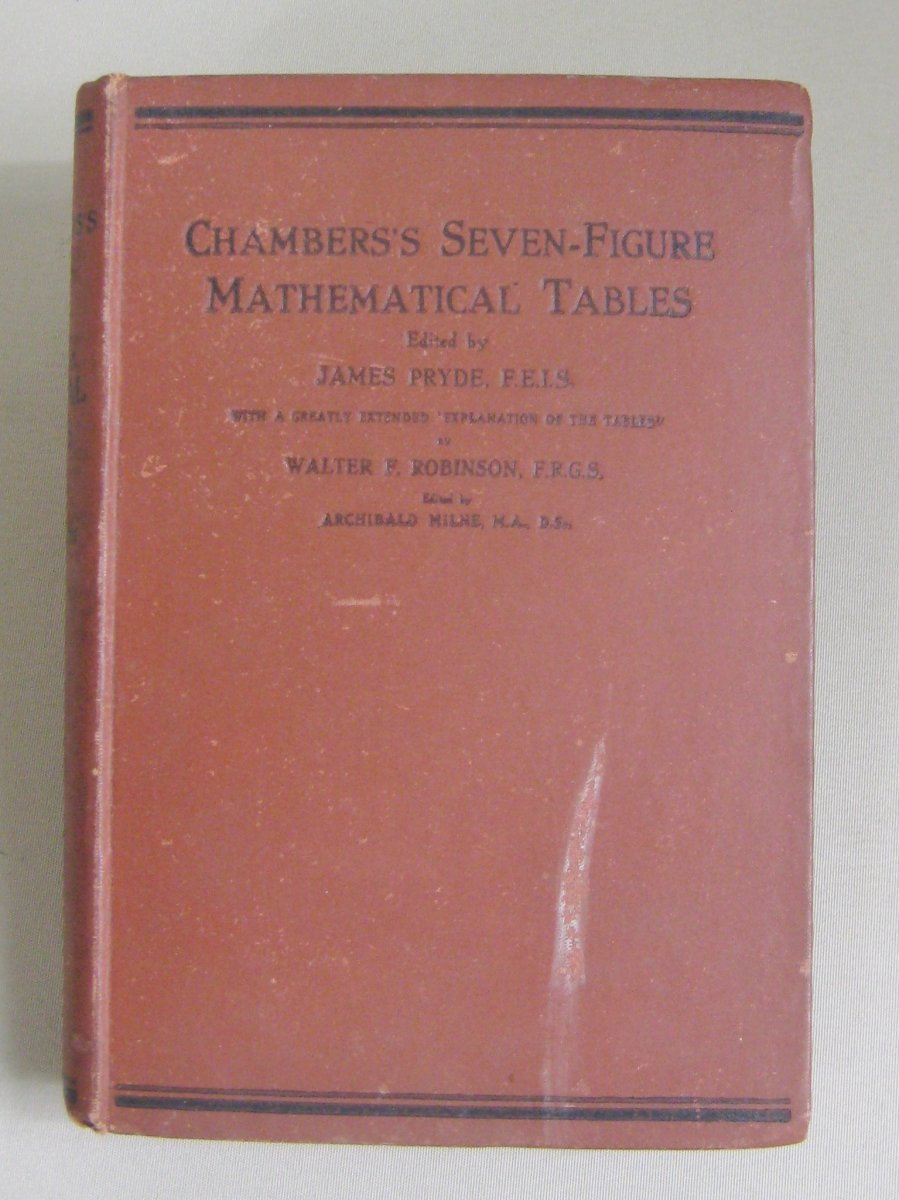•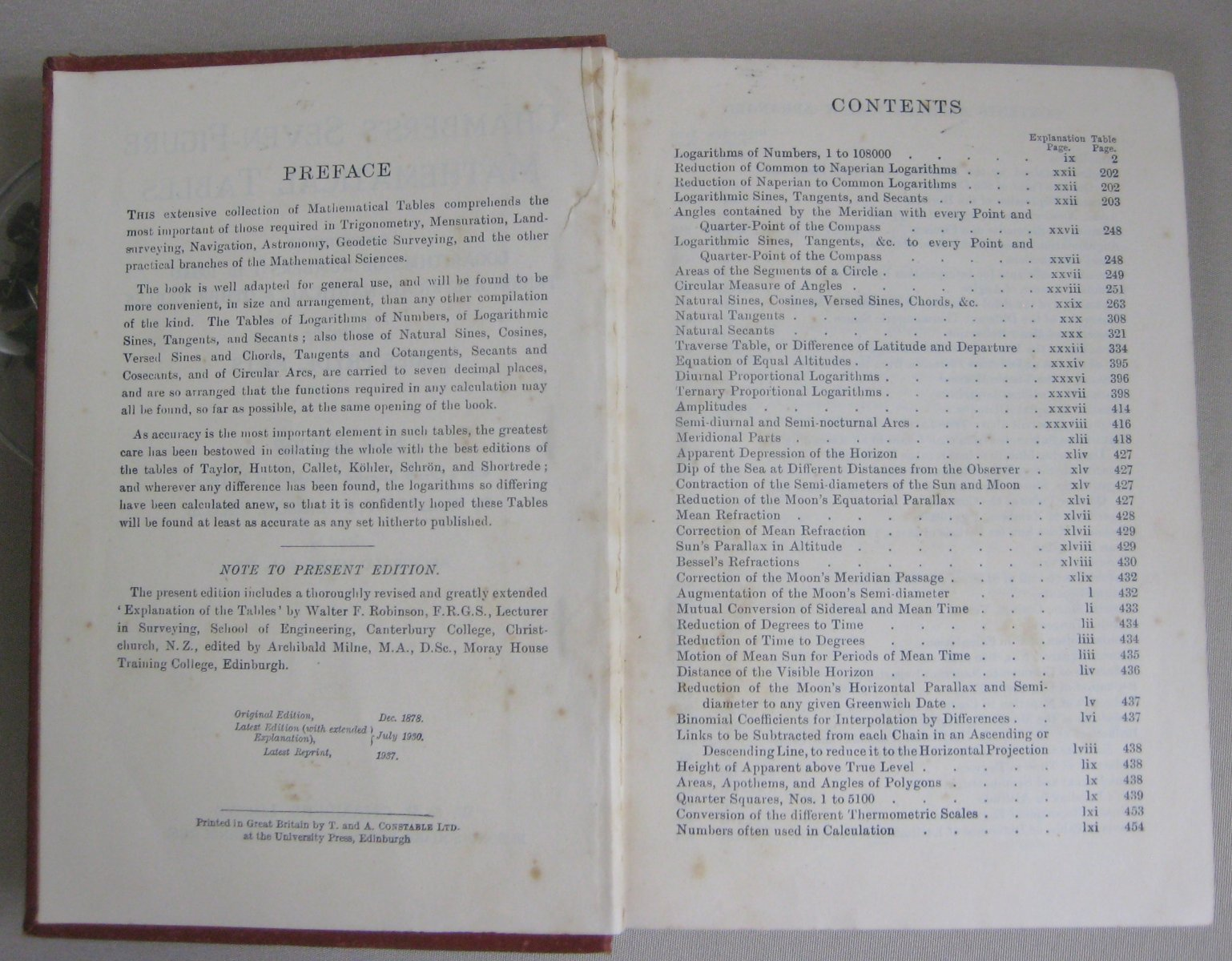•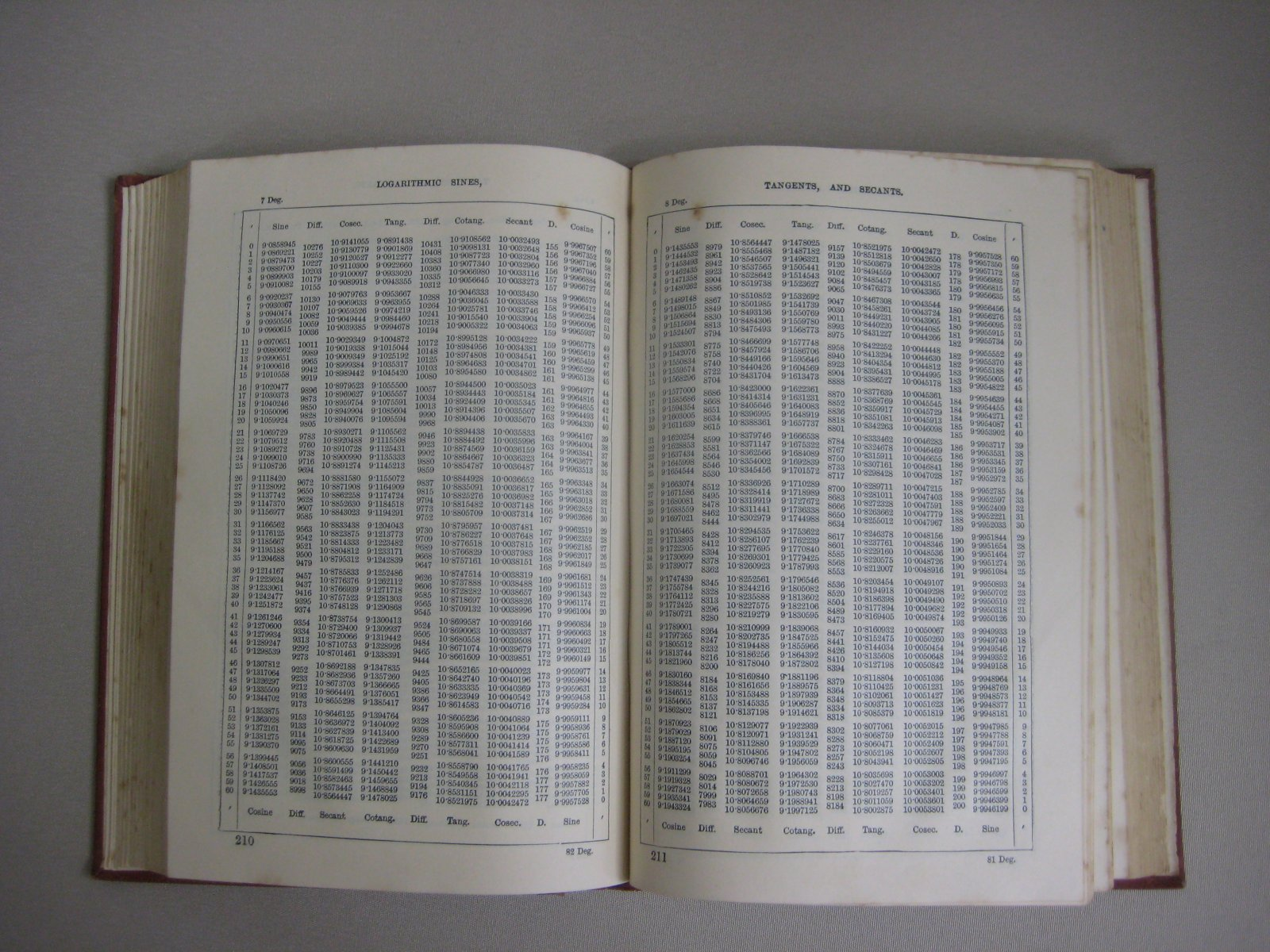•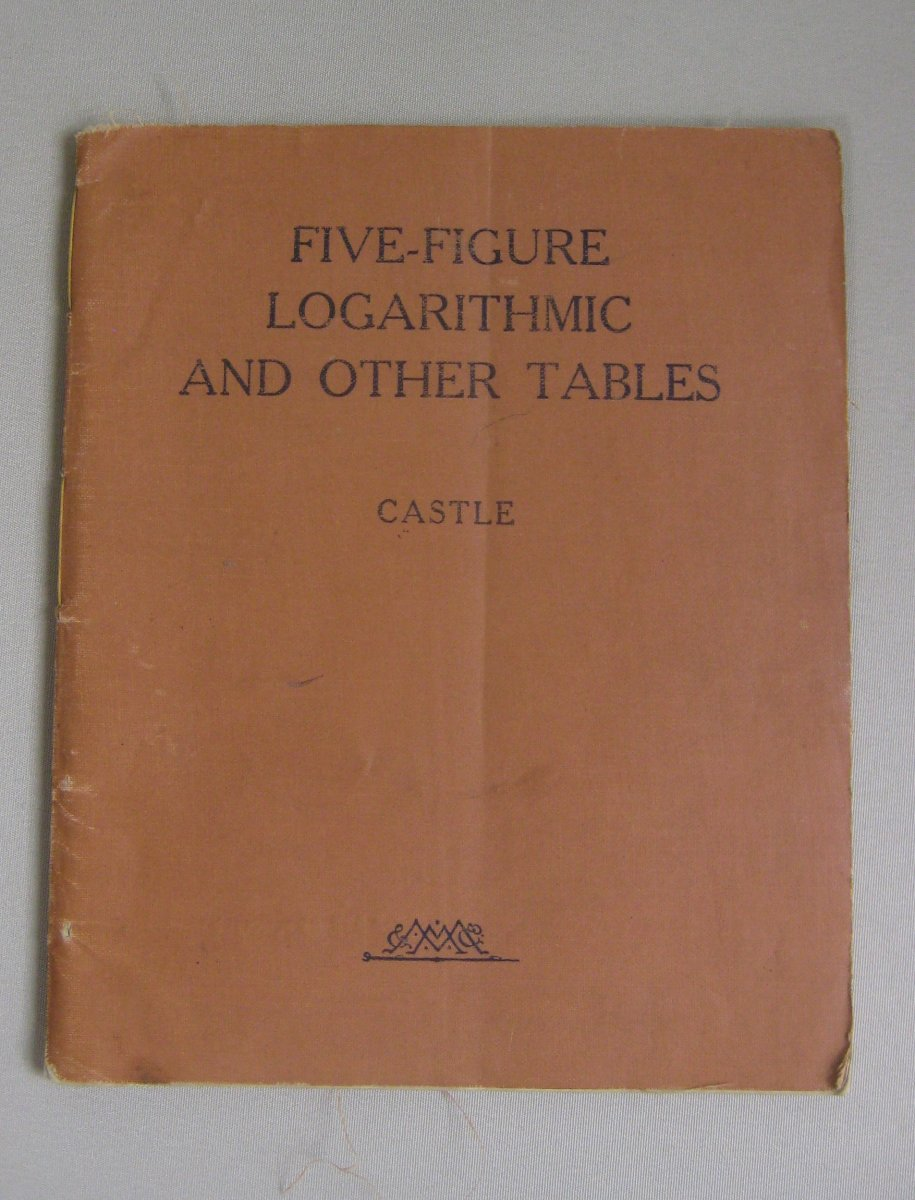•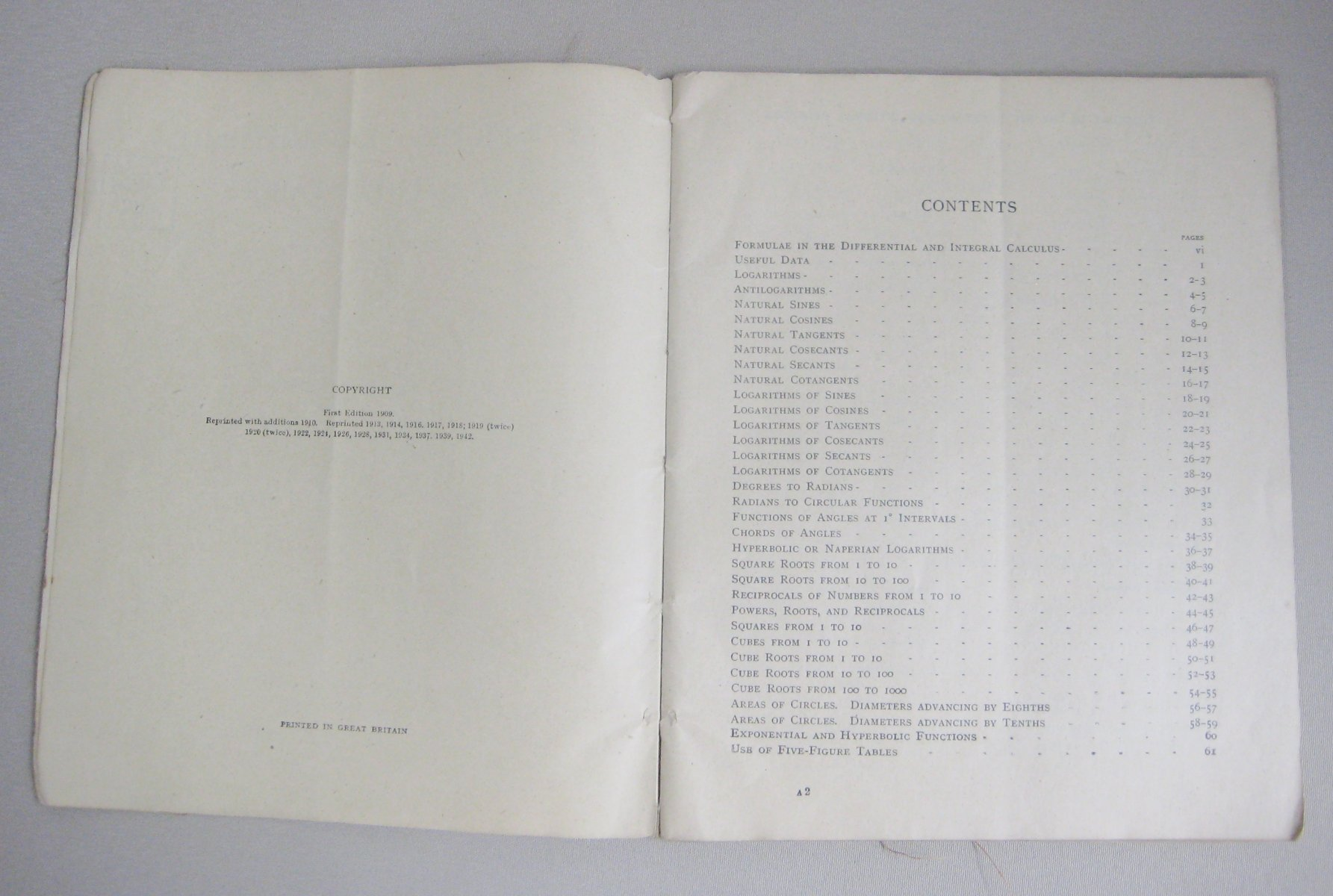•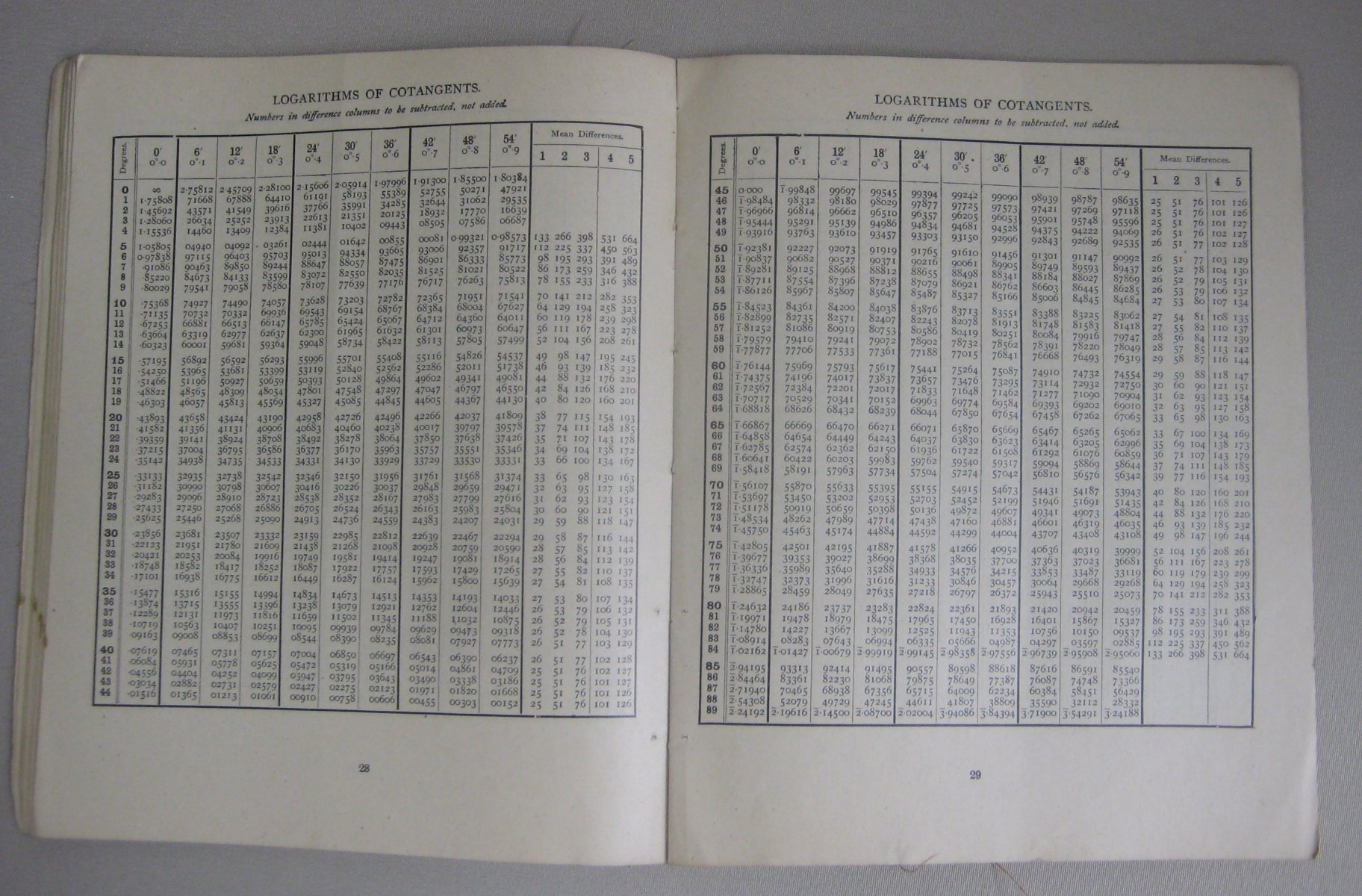••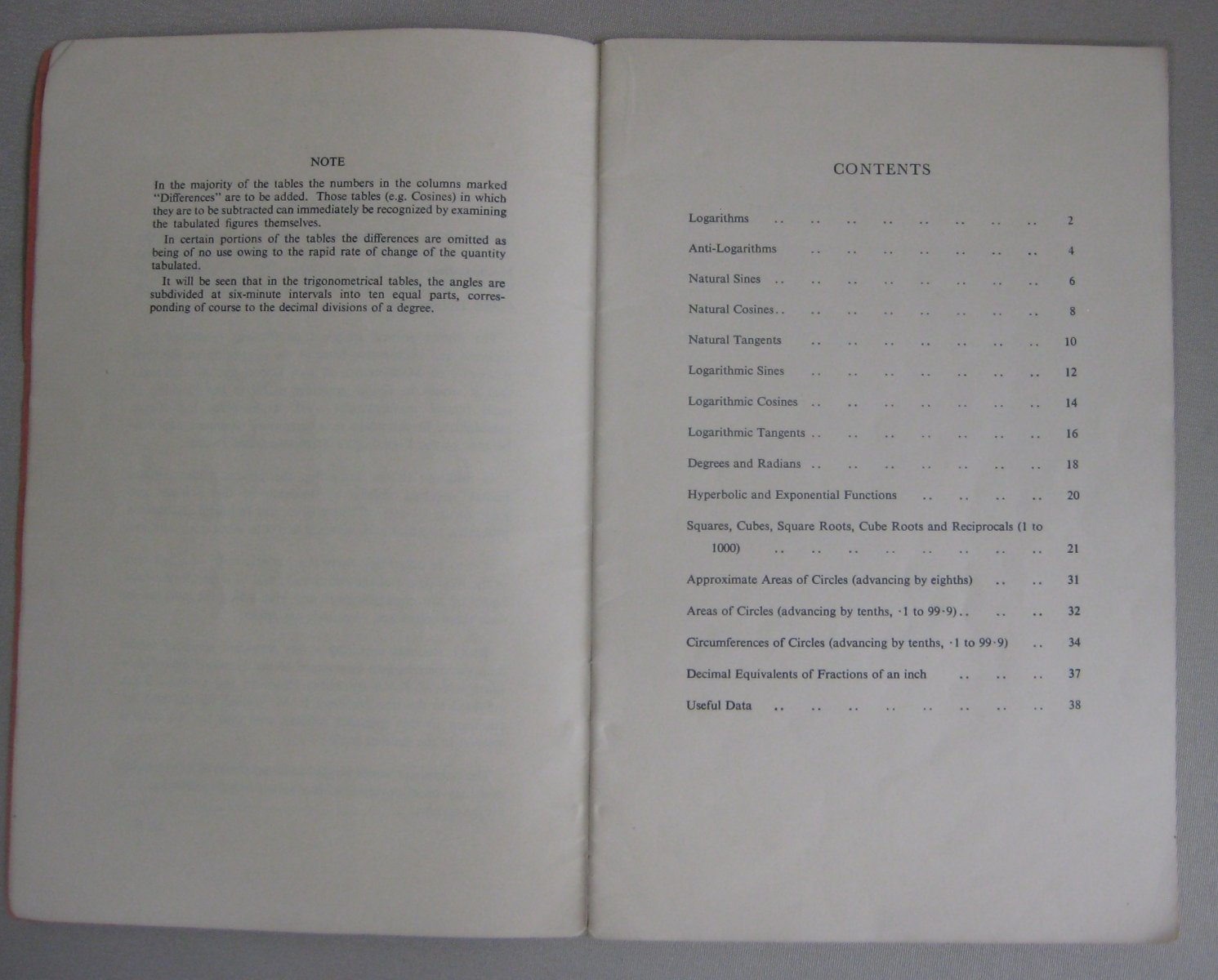•